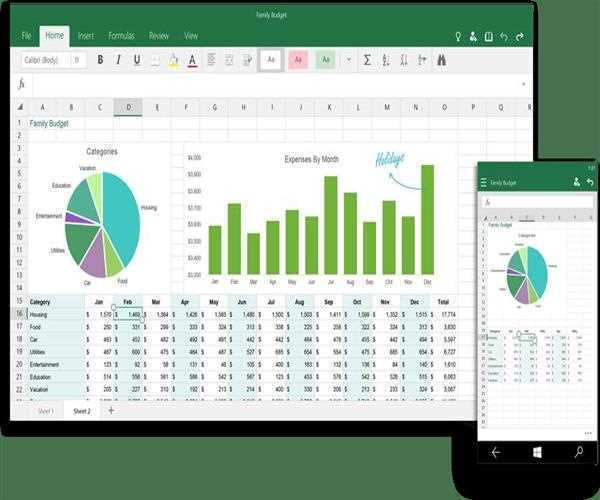# articles

Home / DeveloperSection / Articles / 10 Helpful Excel Formulas759 24-Nov-2020

1. Average. To do the average formula in excel, you have to enter the values, cells, or range of cells you are calculating the average. The formula: =AVERAGE(number1, number1, etc.) or =AVERAGE(Start Value:End Value). It will calculate the average of all the values or ranges of cells included in the parentheses.

2. Percentage. To perform the percentage formula, you have to input the cells in this format, =A1/B1. Highlight the cell, convert the result in a decimal value to that percentage, select 'Percentage' as you click the Home tab, and select 'Percentage' from the dropdown of numbers.

3. PMT. The formula is denoted as =PMT(interest rate, # of periods, present value). This formula is useful for financial analysts working in commercial banking, real estate, any financial task that deals with debt schedules. This formula will give you the value of merely equal payments over the given life of a particular loan.

4. Index. It is an advanced alternative to the VLOOKUP or HLOOKUP formulas. This Index returns a cell's value in a given table based on the desired column and row number.

5. Match. It returns the position of a specified cell in a row or column. Familiarizing the technique using this formula will take your financial analysis and financial modeling to the next level.

6. XPNV. This formula allows you to apply specific dates to each cash flow that is having a discount. Routinely, an analyst tends to assign time evenly on cash flows, so this formula helps you fix it. It is also a lifesaver for those working as a financial analyst in investment banking, equity research, financial planning, and any other corporate finance area that requires discounting cash flows.

7. COUNT. The formula: =COUNT(Start Cell: End Cell). It will return a value equal to the number of entries found within your desired range of cells. It is incredibly helpful for large spreadsheets, wherein you want to see how many cells contain actual entries.

8. SUMIF. The formula: =SUMIF(range, criteria, [sum range]). This formula will help you return the sum of the values within a specified range of cells that meet the same criterion.

9. Trim. The Trim formula is denoted=TRIM(text). It will remove any spaces entered before and after the text entered in the cell.

10. VLOOKUP. This formula is a bit more in-depth than some of the other methods that are helpful when you have two sets of data on two different spreadsheets that you want to combine into a single spreadsheet. It has the formula: VLOOKUP(lookup value, table array, column number, [range lookup]).

Formulas are the heart of spreadsheets; with the right skill handling these formulas, it will allow you to deploy a broad set of razor-sharp tools like these ten helpful excel formulas when solving problems in your organization. Visit eFinancialModels to know other useful excel formulas.

Updated 24-Nov-2020#### Lellith Garcia

Lellith Garcia is the Marketing Manager of eFinancialModels.com, which provides a rich inventory of industry-specific financial model templates in form of Excel spreadsheets. Lellith has been involved in preparing various financial model templates which are loved by entrepreneurs, consultants, investors, and financial analysts who are looking for assistance to speed up their financial modeling tasks.